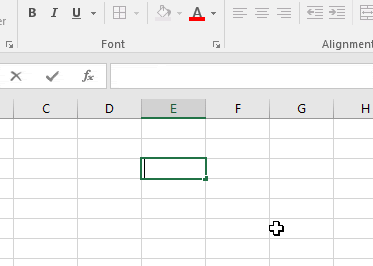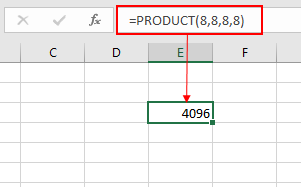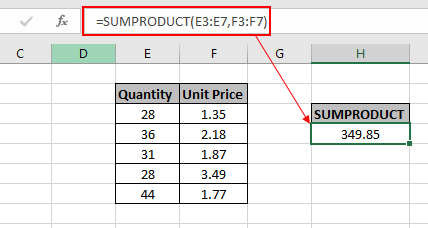# Multiplication in Excel

In this article, we will learn to Multiplication in Excel in Excel.

In simple words, the product of two or more numbers is the SUM of the number of times the other number.

= 2 x 3  (In Mathematics) = 6
= 2 * 3 (In Excel) = 6

There are 3 ways to multiply numbers in Excel.

1. Use the multiply character ( * )
2. Excel PRODUCT function
3. Excel SUMPRODUCT function

Let's learn about them one by one:

Asterisk ( * ) sign is used to multiply numbers same as in mathematics multiply sign does. To use this method first start with equals ( = ) sign  and then use the asterisk ( * ) sign between every number, to multiply.As shown in the above gif that 8 times into 8 times into 8 times 8 is equal to 4096.

Second method is using the Excel mathematical formula, The PRODUCT function

The PRODUCT function is a mathematical operator function which multiplies the numbers. PRODUCT function excel returns product of all cell values taken in as arguments.

Syntax:

= PRODUCT (number1, [number2], ...)As you can see from the above snapshot that product function returns the same result as the excel Multiply character ( * ) does.

Now we will see the third method which is the SUMPRODUCT function which first takes the product and then returns the SUM.

SUMPRODUCT function is a mathematical function in Excel. It operates on multiple ranges. It multiplies the corresponding arrays and then adds them.

Total= ( A1 * B1 * C1 * .. + A2 * B2 * C2 * ..  + …)

Syntax:

= SUMPRODUCT (array1, [array2], ...)As you can see from the snapshot that we got the total Price for the data without calculating in individual rows.

Notes:

1. The SUMPRODUCT function considers non - numeric values as 0s.
2. The SUMPRODUCT function considers logic value TRUE as 1 and False as 0.
3. The argument array must be of same length else the function returns error.

Hope this article about how to Multiplication in Excel in Excel is explanatory. Find more articles on SUMPRODUCT functions here. Please share your query below in the comment box. We will assist you.

Related Articles

How to use the SUMPRODUCT function in Excel

How to use the PRODUCT function in Excel

Validation of text entries

Create drop down list in excel with colour

Remove leading and trailing spaces from text in Excel

Popular Articles

50 Excel Shortcut to Increase Your Productivity

Edit a dropdown list

Absolute reference in Excel

If with conditional formatting

If with wildcards

Vlookup by date

Convert Inches To Feet and Inches in Excel 2016

Join first and last name in excel

Count cells which match either A or B

Terms and Conditions of use

The applications/code on this site are distributed as is and without warranties or liability. In no event shall the owner of the copyrights, or the authors of the applications/code be liable for any loss of profit, any problems or any damage resulting from the use or evaluation of the applications/code.